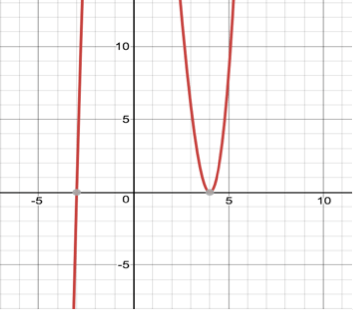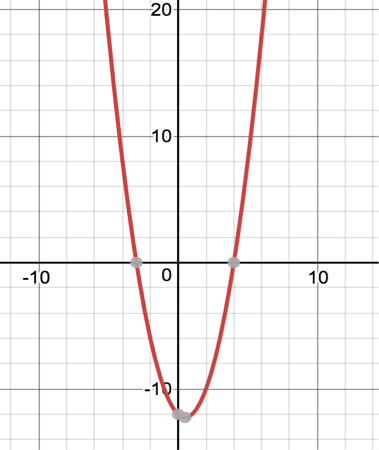# POLQ 3 | Lesson 3 | Explore (Understanding Multiplicity)

The term multiplicity refers to the number of times a polynomial has the same zero. There are four graphs with the same rational zeros, -3 and 4. However some of the zeros have multiplicity of one and others of two. See if you can match which each graph with the appropriate polynomial function without the use of a graphing calculator.

1)2)3)4)s

a) $$y=(x+3)(x-4)^4$$

b) $$y=(x+3)^2(x-4)^2$$

c) $$y=(x+3)(x-4)$$

d) $$y=(x+3)^2(x-4)$$

##### Making Connections (Multiplicity)

Check solutions here.